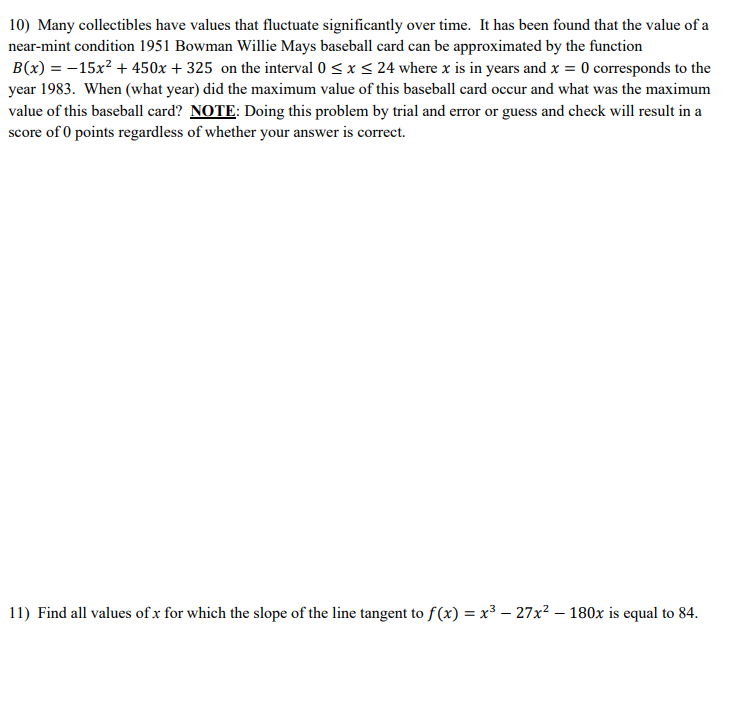# Question 10) Many collectibles have values that fluctuate significantly over time. It has been found that the value of a near-mint condition 1951 Bowman Willie Mays baseball card can be approximated by the function B(x) = -15x² + 450x + 325 on the interval 0 ≤ x ≤ 24 where x is in years and x = 0 corresponds to the year 1983. When (what year) did the maximum value of 10) Many collectibles have values that fluctuate significantly over time. It has been found that the value of a near-mint condition 1951 Bowman Willie Mays baseball card can be approximated by the function B(x) = -15x² + 450x + 325 on the interval 0 ≤ x ≤ 24 where x is in years and x = 0 corresponds to the year 1983. When (what year) did the maximum value of this baseball card occur and what was the maximum value of this baseball card? NOTE: Doing this problem by trial and error or guess and check will result in a score of 0 points regardless of whether your answer is correct. 11) Find all values of x for which the slope of the line tangent to f(x) = x³ - 27x² - 180x is equal to 84.GFBEON The Asker · Other MathematicsTranscribed Image Text: 10) Many collectibles have values that fluctuate significantly over time. It has been found that the value of a near-mint condition 1951 Bowman Willie Mays baseball card can be approximated by the function B(x) = -15x² + 450x + 325 on the interval 0 ≤ x ≤ 24 where x is in years and x = 0 corresponds to the year 1983. When (what year) did the maximum value of this baseball card occur and what was the maximum value of this baseball card? NOTE: Doing this problem by trial and error or guess and check will result in a score of 0 points regardless of whether your answer is correct. 11) Find all values of x for which the slope of the line tangent to f(x) = x³ - 27x² - 180x is equal to 84.
More
Transcribed Image Text: 10) Many collectibles have values that fluctuate significantly over time. It has been found that the value of a near-mint condition 1951 Bowman Willie Mays baseball card can be approximated by the function B(x) = -15x² + 450x + 325 on the interval 0 ≤ x ≤ 24 where x is in years and x = 0 corresponds to the year 1983. When (what year) did the maximum value of this baseball card occur and what was the maximum value of this baseball card? NOTE: Doing this problem by trial and error or guess and check will result in a score of 0 points regardless of whether your answer is correct. 11) Find all values of x for which the slope of the line tangent to f(x) = x³ - 27x² - 180x is equal to 84.